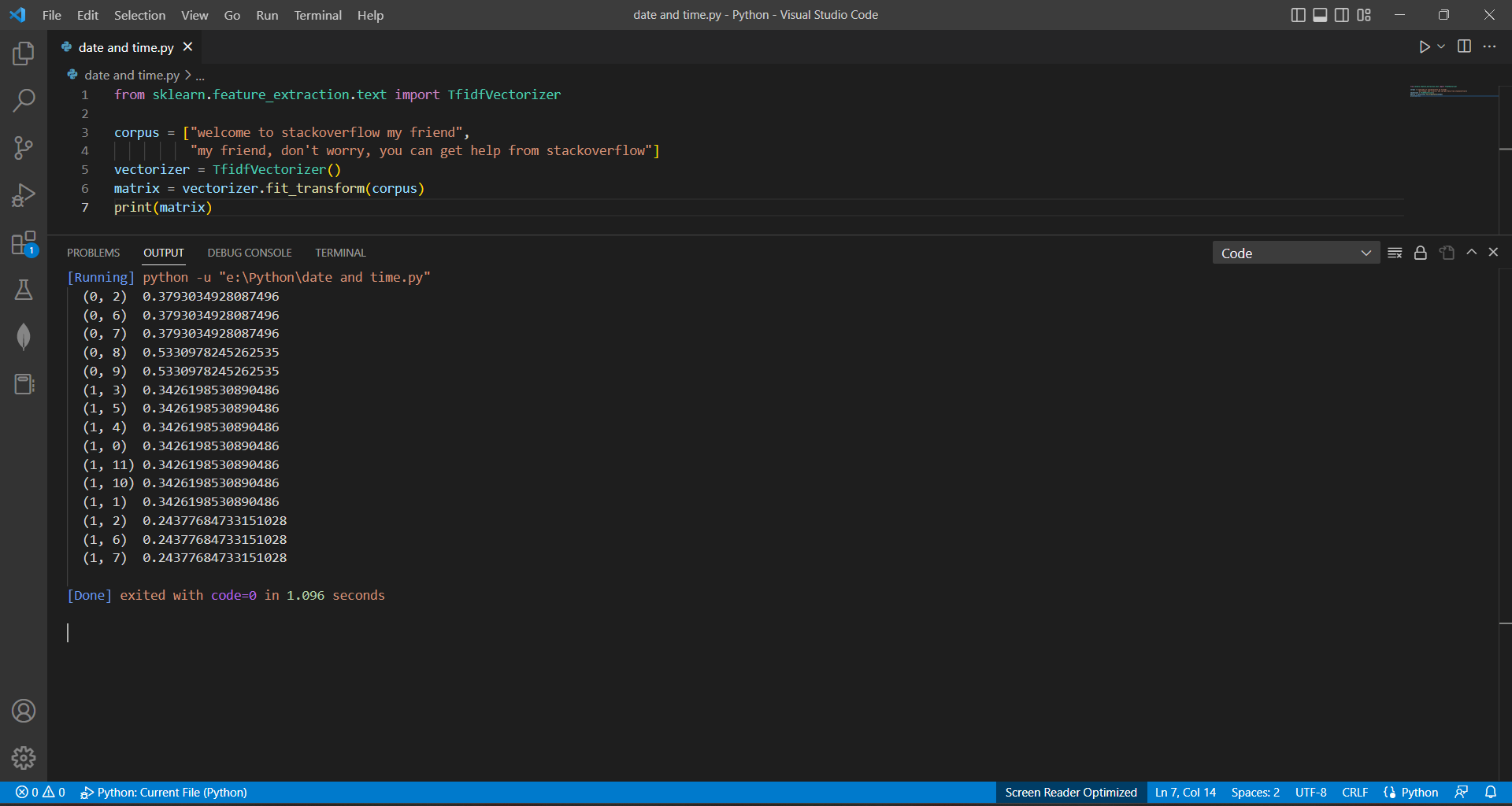# How to Create Tf-idf Matrixby vigneshchennai74Updated: Feb 9, 2023Solution Kit

To create a TF-IDF vectorizer in SKlearn, you can use the TfidfVectorizer class from the sklearn.feature_extraction.text module. the main use of a TF-IDF vectorizer is to convert text data into numerical features that can be used as input to machine learning algorithms for various NLP and IR tasks.

TF-IDF (Term Frequency-Inverse Document Frequency) is a numerical representation of the importance of each word in a corpus of text documents. It is commonly used as a feature representation for text data in various natural language processing (NLP) and information retrieval (IR) tasks. The use of a TF-IDF vectorizer is to convert a collection of raw text documents into numerical features that can be used as input to machine learning algorithms. The resulting TF-IDF matrix provides a representation of the importance of each word in each document, relative to the importance of that word across the entire corpus.

By transforming the text data into a numerical representation, the TF-IDF vectorizer enables the use of machine learning algorithms that otherwise would not be able to work with text data. This can include various classification, clustering, and dimensionality reduction algorithms. In addition, the TF-IDF vectorizer also helps to reduce the dimensionality of the data, which is important when working with high-dimensional data, as well as to remove stop words and perform other text pre-processing tasks.

The TfidfVectorizer is initialized and then fit into the corpus of text documents. The fit_transform method is used to fit the vectorizer to the corpus and to transform the corpus into a TF-IDF matrix at the same time. The resulting matrix is a sparse matrix representation of the TF-IDF values for each term in the corpus.

Here is the example of how to create Tf-idf Vectonizer:Preview of the output that you will get on running this code from your IDE

### Code

In this solution we have used TfidfVectorizer .

1. Copy the code using the "Copy" button above, and paste it in a Python file in your IDE.
2. Run the file to get the output

I hope you found this useful. I have added the link to dependent libraries, version information in the following sections.

I found this code snippet by searching for "Creating a TF-IDF Matrix Python 3.6" in kandi. You can try any such use case!

Note

If the user needs to print the words and their index use this command

for i, feature in enumerate(vectorizer.get_feature_names()):

print(i, feature)

or

Copy from our code snippet

### Environment Tested

I tested this solution in the following versions. Be mindful of changes when working with other versions.

1. The solution is created in Python 3.7.15 version
2. The solution is tested on scikit-learn 1.0.2 version

Using this solution, we are able going to learn how to Creating Tf-idf Matrix and calculating the weight a matrix using Scikit learn library in Python with simple steps. This process also facilities an easy to use, hassle free method to create a hands-on working version of code which would help Creating Tf-idf Matrix and calculating the weight in Python.

### Dependent Library

scikit-learnby scikit-learn

Python54584Version:1.2.2scikit-learn: machine learning in Python

Support
Quality
Security
Reuse

scikit-learnby scikit-learn

Python54584Version:1.2.2License: Permissive (BSD-3-Clause)

scikit-learn: machine learning in Python
Support
Quality
Security